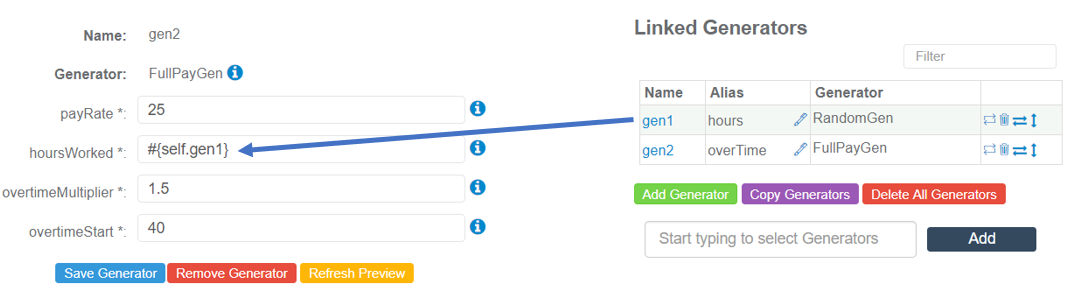# Description

The FullPayGen Generator computes regular pay plus overtime pay.

## Parameters

The following parameters may be configured for the FullPayGen Generator. Items with an asterisk* are required.

• payRate* - Defines the rate of pay that is given per hour. The default is 25.

• hoursWorked* - Defines the total number of hours worked. The default is 50.

• overtimeMultiplier* - Defines the rate at which overtime should be multiplied and calculated. The default is 1.5, which is time and a half.

• overtimeStart* - Defines the amount of hours worked after which the overtime should be calculated. The default is 40 hours.## Example 1: Generate Hours Between 1 and 100 and Use for Full Pay Calculation

For this example, the RandomGen Generator has been linked to the FullPayGen Generator to generate a number of hours between 1 and 100, which will be used to calculate pay.

RandomGen Generator (gen1) is referenced within the hoursWorked Parameter, as shown below:The RandomGen Generator (gen1) configuration is shown below:## Sample Output